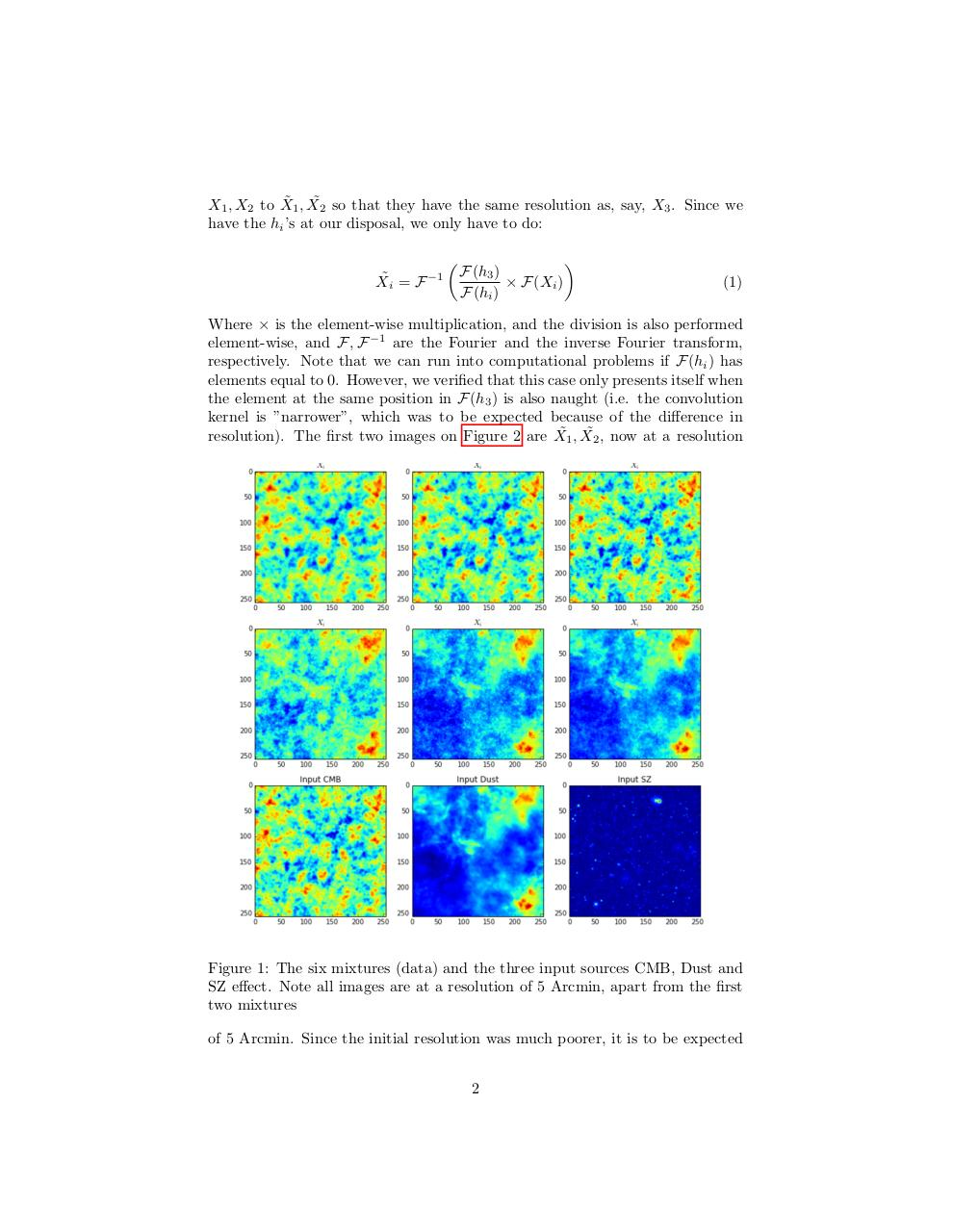# Report SchmitzSitbon.pdfPage 1 2 3 4 5 6 7 8 9 10

#### Text preview

˜ 1 , X˜2 so that they have the same resolution as, say, X3 . Since we
X1 , X2 to X
have the hi ’s at our disposal, we only have to do:

X˜i = F −1



F(h3 )
× F(Xi )
F(hi )


(1)

Where × is the element-wise multiplication, and the division is also performed
element-wise, and F, F −1 are the Fourier and the inverse Fourier transform,
respectively. Note that we can run into computational problems if F(hi ) has
elements equal to 0. However, we verified that this case only presents itself when
the element at the same position in F(h3 ) is also naught (i.e. the convolution
kernel is ”narrower”, which was to be expected because of the difference in
resolution). The first two images on Figure 2 are X˜1 , X˜2 , now at a resolution

Figure 1: The six mixtures (data) and the three input sources CMB, Dust and
SZ effect. Note all images are at a resolution of 5 Arcmin, apart from the first
two mixtures
of 5 Arcmin. Since the initial resolution was much poorer, it is to be expected
2